# A Global Optimization Algorithm for Sparse Mixed Membership Matrix Factorization

• 696 Accesses

Part of the ICSA Book Series in Statistics book series (ICSABSS)

## Abstract

Mixed membership factorization is a popular approach for analyzing data sets that have within-sample heterogeneity. In recent years, several algorithms have been developed for mixed membership matrix factorization, but they only guarantee estimates from a local optimum. Here, we derive a global optimization algorithm that provides a guaranteed 𝜖-global optimum for a sparse mixed membership matrix factorization problem. We test the algorithm on simulated data and a small real gene expression dataset and find the algorithm always bounds the global optimum across random initializations and explores multiple modes efficiently.

This is a preview of subscription content, access via your institution.

Chapter
USD   29.95
Price excludes VAT (USA)
• DOI: 10.1007/978-3-030-15310-6_7
• Chapter length: 28 pages
• Own it forever
• Exclusive offer for individuals only
• Tax calculation will be finalised during checkout
eBook
USD   109.00
Price excludes VAT (USA)
• ISBN: 978-3-030-15310-6
• Own it forever
• Exclusive offer for individuals only
• Tax calculation will be finalised during checkout
Softcover Book
USD   139.00
Price excludes VAT (USA)
Hardcover Book
USD   179.99
Price excludes VAT (USA)

## References

• Airoldi, E.M., Blei, D.M., Fienberg, S.E., Xing, E.P.: Mixed membership stochastic blockmodels. J. Mach. Learn. Res. 9, 1981–2014 (2008)

• Benders, J.F.: Partitioning procedures for solving mixed-variables programming problems. Numer. Math. 4(1), 238–252 (1962)

• Blei, D.M., Lafferty, J.D.: Correlated topic models. In: Proceedings of the International Conference on Machine Learning, pp 113–120 (2006)

• Blei, D.M., Ng, A.Y., Jordan, M.I.: Latent dirichlet allocation. J. Mach. Learn. Res. 3, 993–1022 (2003)

• Blei, D.M., Kucukelbir, A., McAuliffe, J.D.: Variational inference: a review for statisticians. J. Am. Stat. Assoc. 112(518), 859–877 (2017)

• Boyd, S., Vandenberghe, L.: Convex Optimization. Cambridge University Press, Cambridge (2004)

• Dheeru, D., Karra T.E.: UCI machine learning repository. URL UCI machine learning repository (2017). http://archive.ics.uci.edu/ml

• Floudas, C.A.: Deterministic Global Optimization, Nonconvex Optimization and Its Applications, vol 37. Springer, Boston (2000)

• Floudas, C.A.: Deterministic Global Optimization: Theory, Methods and Applications, vol. 37. Springer, Berlin (2013)

• Floudas, C.A., Gounaris, C.E.: A review of recent advances in global optimization. J. Glob. Optim. 45, 3–38 (2008)

• Floudas, C.A., Visweswaran, V.: A global optimization algorithm (GOP) for certain classes of nonconvex NLPS. Comput. Chem. Eng. 14(12), 1–34 (1990)

• Geoffrion, A.M.: Generalized benders decomposition. J. Optim. Theory Appl. 10, 237–260 (1972)

• Gorski, J., Pfeuffer, F., Klamroth, K.: Biconvex sets and optimization with biconvex functions: a survey and extensions. Math. Methods Oper. Res. 66, 373–407 (2007)

• Gurobi Optimization, Inc (2018) Gurobi optimizer version 8.0

• Horst, R., Tuy, H.: Global Optimization: Deterministic Approaches. Springer, Berlin (2013)

• Kabán, A.: On Bayesian classification with laplace priors. Pattern Recognit. Lett. 28(10), 1271–1282 (2007)

• Lancaster, P., Tismenetsky, M., et al.: The theory of matrices: with applications. Elsevier, San Diego (1985)

• Lee, D.D., Seung, H.S.: Learning the parts of objects by non-negative matrix factorization. Nature 401, 788–791 (1999)

• MacKay, D.J.C.: Bayesian interpolation. Neural Comput. 4(3), 415–447 (1992)

• Mackey, L., Weiss, D., Jordan, M.I.: Mixed membership matrix factorization. In: International Conference on Machine Learning, pp 1–8 (2010)

• Pritchard, J.K., Stephens, M., Donnelly, P.: Inference of population structure using multilocus genotype data. Genetics 155, 945–959 (2000)

• Saddiki, H., McAuliffe, J., Flaherty, P.: GLAD: a mixed-membership model for heterogeneous tumor subtype classification. Bioinformatics 31(2), 225–232 (2015)

• Singh, A.P., Gordon, G.J.: A unified view of matrix factorization models. In: Lecture Notes in Computer Science, vol. 5212, pp. 358–373, Springer, Berlin (2008)

• Taddy, M.: Multinomial inverse regression for text analysis. J. Am. Stat. Assoc. 108(503), 755–770, (2013). https://doi.org/10.1080/01621459.2012.734168

• Teh, Y.W., Jordan, M.I., Beal, M.J., Blei, D.M.: Sharing clusters among related groups: hierarchical Dirichlet processes. In: Advances in Neural Information Processing Systems, vol. 1, MIT Press, Cambridge (2005)

• Weinstein, J.N., Collisson, E.A., Mills, G.B., Shaw, K.R.M., Ozenberger, B.A., Ellrott, K., Shmulevich, I., Sander, C., Stuart, J.M., Network CGAR, et al.: The cancer genome atlas pan-cancer analysis project. Nat. Genet. 45(10), 1113 (2013)

• Xiao, H., Stibor, T.: Efficient collapsed Gibbs sampling for latent Dirichlet allocation. In: Sugiyama, M., Yang, Q. (eds.) Proceedings of 2nd Asian Conference on Machine Learning, vol. 13, pp. 63–78 (2010)

• Xu, W., Liu, X., Gong, Y.: Document clustering based on non-negative matrix factorization. In: Proceedings of the 26th Annual International ACM SIGIR Conference on Research and Development in Information Retrieval–SIGIR ’03, p. 267 (2003)

• Zaslavsky, T.: Facing Up to Arrangements: Face-Count Formulas for Partitions of Space by Hyperplanes: Face-Count Formulas for Partitions of Space by Hyperplanes, vol. 154. American Mathematical Society (1975)

## Acknowledgements

We acknowledge Hachem Saddiki for valuable discussions and comments on the manuscript.

## Author information

Authors

### Corresponding author

Correspondence to Patrick Flaherty .

## Appendices

### Appendix 1: Derivation of Relaxed Dual Problem Constraints

The Lagrange function is the sum of the Lagrange functions for each sample,

\displaystyle \begin{aligned} L(y, \theta, x, \lambda) = \sum_{i=1}^n L(y_i, \theta_i, x, \lambda_i, \mu_i), \end{aligned}
(7.12)

and the Lagrange function for a single sample is

\displaystyle \begin{aligned} L(y_i, \theta_i, x, \lambda_i, \mu_i) = y_i^T y_i -2 y_i^T x\theta_i + \theta_i^T x^T x \theta_i - \lambda_i(\theta_i^T 1_K - 1) -\mu_i^T \theta_i. \end{aligned}
(7.13)

We see that the Lagrange function is biconvex in x and θ i. We develop the constraints for a single sample for the remainder.

### 7.1.1 Linearized Lagrange Function with Respect to x

Casting x as a vector and rewriting the Lagrange function gives

\displaystyle \begin{aligned} L(y_i, \theta_i, \bar{x}, \lambda_i, \mu_i) = a_i - 2b_i^T\bar{x} + \bar{x}^TC_i\bar{x} - \lambda_i(\theta_i^T 1_K - 1) -\mu_i^T\theta_i, \end{aligned}
(7.14)

where $$\bar {x}$$ is formed by stacking the columns of x in order. The coefficients are formed such that

\displaystyle \begin{aligned} \begin{array}{rcl} a &\displaystyle =&\displaystyle y_i^T y_i, \\ b_i^T \bar{x} &\displaystyle =&\displaystyle y_i^T x \theta_i, \\ \bar{x}^T C_i \bar{x} &\displaystyle =&\displaystyle \theta_i^T x^T x \theta_i. \end{array} \end{aligned}

The linear coefficient matrix is the KM × 1 vector,

\displaystyle \begin{aligned} b_i = \left[ y_{i}\theta_{1i}, \cdots, y_{i}\theta_{Ki} \right] \end{aligned}

The quadratic coefficient is the KM × KM and block matrix

\displaystyle \begin{aligned} C_i = \left[ \begin{array}{ccc} \theta^2_{1i} I_M & \cdots & \theta_{1i} \theta_{Ki} I_M \\ \vdots & \ddots & \vdots \\ \theta_{Ki} \theta_{1i} I_M & \cdots & \theta^2_{Ki} I_M \end{array} \right] \end{aligned}

The Taylor series approximation about x 0 is

\displaystyle \begin{aligned} L(y_i, \theta_i, \bar{x}, \lambda_i, \mu_i) \bigg|{}^{\text{lin}}_{\bar{x}_0} = L(y_i, x_0, \theta_i, \lambda_i, \mu_i) + (\nabla_x L |{}_{x_0})^T(x-x_0). \end{aligned}
(7.15)

The gradient with respect to x is

\displaystyle \begin{aligned} \nabla_x L(y_i, \theta_i, \bar{x}, \lambda_i, \mu_i) = -2 b_i + 2 C_i \bar{x}. \end{aligned}
(7.16)

Plugging the gradient into the Taylor series approximation gives

\displaystyle \begin{aligned} L(y_i, \theta_i, \bar{x}, \lambda_i) \bigg|{}^{\text{lin}}_{\bar{x}_0} {=} a_i - 2b_i^T\bar{x}_0 + \bar{x}_0^TC_i\bar{x}_0 - \lambda_i\left(\theta_i^T 1_K - 1\right) - \mu_i^T \theta_i + (-2 b_i + 2 C_i \bar{x}_0)^T(\bar{x}-\bar{x}_0). \end{aligned}
(7.17)

Simplifying the linearized Lagrange function gives

\displaystyle \begin{aligned} L(y_i, \theta_i, \bar{x}, \lambda_i, \mu_i) \bigg|{}^{\text{lin}}_{\bar{x}_0} = \left(y_i^T y_i - \bar{x}_0^T C_i \bar{x}_0 - \lambda_i\left(\theta_i^T 1_K - 1\right) - \mu_i^T \theta_i\right) - 2 b_i^T \bar{x} + 2 \bar{x}_0^T C_i \bar{x} \end{aligned}
(7.18)

Finally, we write the linearized Lagrangian using the matrix form of x 0,

\displaystyle \begin{aligned} L(y_i, \theta_i, x, \lambda_i, \mu_i) \bigg|{}^{\text{lin}}_{x_0} = y_i^T y_i^T - \theta_i^T x_0^T x_0 \theta_i - 2 y_i^T x \theta_i + 2 \theta_i^T x_0^T x \theta_i - \lambda_i\left(\theta_i^T 1_K - 1\right) - \mu_i^T \theta_i \end{aligned}
(7.19)

While the original Lagrange function is convex in θ i for a fixed x, the linearized Lagrange function is not necessarily convex in θ i. This can be seen by collecting the quadratic, linear and constant terms with respect to θ i,

\displaystyle \begin{aligned} L(y_i, \theta_i, x, \lambda_i, \mu_i) \bigg|{}^{\text{lin}}_{x_0} = \left(y_i^T y_i^T + \lambda_i\right) + \left(- 2 y_i^T x -\lambda_i 1_K^T -\mu_i^T \right) \theta_i + \theta_i^T \left(2 x_0^T x - x_0^T x_0 \right) \theta_i. \end{aligned}
(7.20)

Now, if and only if $$2x_0^Tx - x_0^Tx_0 \succeq 0$$ is positive semidefinite, then $$L(y_i, \theta _i, x, \lambda _i, \mu _i) \bigg |{ }^{\text{lin}}_{x_0}$$ is convex. The condition is satisfied at x = x 0 but may be violated at some other value of x.

### 7.1.2 Linearized Lagrange Function with Respect to θi

Now, we linearize (7.18) with respect to θ i. Using the Taylor series approximation with respect to θ 0i gives

\displaystyle \begin{aligned} L(y_i, \theta_i, x, \lambda_i, \mu_i) \bigg|{}^{\text{lin}}_{x_0, \theta_{0i}} = L(y_i, \theta_{0i}, x, \lambda_i, \mu_i) \bigg|{}^{\text{lin}}_{x_0} + \left( \nabla_{\theta_i} L(y_i, \theta_i, x, \lambda_i, \mu_i) \bigg|{}^{\text{lin}}_{x_0} \bigg|{}_{\theta_{0i}} \right)^T (\theta_i - \theta_{0i}) \end{aligned}
(7.21)

The gradient for this Taylor series approximation is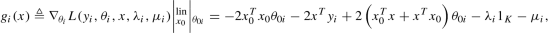(7.22)

where g i(x) is the vector of K qualifying constraints associated with the Lagrange function. The qualifying constraint is linear in x. Plugging the gradient into the approximation gives

\displaystyle \begin{aligned} \begin{gathered} L(y_i, \theta_i, x, \lambda_i, \mu_i) \bigg|{}^{\text{lin}}_{x_0, \theta_{0i}} = y_i^T y_i^T - \theta_{0i}^T x_0^T x_0 \theta_{0i} - 2 y_i^T x \theta_{0i} + 2 \theta_{0i}^T x_0^T x \theta_{0i} - \lambda_i\left(\theta_{0i}^T 1_K - 1\right)\\ \quad - \mu_i^T \theta_{0i} + \left(-2 x_0^T x_0 \theta_{0i} -2 x^T y_i + 2 (x_0^T x + x^T x_0) \theta_{0i} - \lambda_i 1_K - \mu_i \right)^T (\theta_i - \theta_{0i}) \end{gathered} \end{aligned}
(7.23)

The linearized Lagrange function is bi-linear in x and θ i. Finally, simplifying the linearized Lagrange function gives

\displaystyle \begin{aligned} \begin{aligned} L(y_i, \theta_i, x, \lambda_i, \mu_i) \bigg|{}^{\text{lin}}_{x_0, \theta_{0i}} =\ & y_i^T y_i^T + \theta_{0i}^T x_0^T x_0 \theta_{0i} -2 \theta_{0i}^T x_0^T x_0 \theta_i - \lambda_i(1_K^T \theta_i - 1) - \mu_i^T \theta_i \\ & - 2 \theta_{0i}^T x^T x_0 \theta_{0i} - 2 y_i^T x \theta_i + 2 \theta_{0i}^T (x_0^T x + x^T x_0) \theta_i \end{aligned} \end{aligned}
(7.24)

### Appendix 2: Proof of Biconvexity

To prove the optimization problem is biconvex, first we show the feasible region over which we are optimizing is biconvex. Then, we show the objective function is biconvex by fixing θ and showing convexity with respect to x, and then vice versa.

### 7.1.1 The Constraints Form a Biconvex Feasible Region

Our constraints can be written as

\displaystyle \begin{aligned} ||x||{}_1 & \leqslant P {} \end{aligned}
(7.25)
\displaystyle \begin{aligned} \sum_{k=1}^{K}\theta_{ki} & = 1 \ \forall i {} \end{aligned}
(7.26)
\displaystyle \begin{aligned} 0 \leqslant \theta_{ki} & \leqslant 1 \ \forall (k, i). {} \end{aligned}
(7.27)

The inequality constraint (7.25) is convex if either x or θ is fixed, because any norm is convex. The equality constraints (7.26) is an affine combination that is still affine if either x or θ is fixed. Every affine set is convex. The inequality constraint (7.27) is convex if either x or θ is fixed, because θ is a linear function.

### 7.1.2 The Objective Is Convex with Respect to θ

We prove the objective is a biconvex function using the following two theorems.

### Theorem 1

Let $$A \subseteq {\mathbb {R}^n}$$ be a convex open set and let $$f: A \rightarrow \mathbb {R}$$ be twice differentiable. Write H(x) for the Hessian matrix of f at x  A. If H(x) is positive semidefinite for all x  A, then f is convex (Boyd and Vandenberghe 2004).

### Theorem 2

A symmetric matrix A is positive semidefinite (PSD) if and only if there exists B such that A = B TB (Lancaster et al. 1985).

The objective of our problem is,

\displaystyle \begin{aligned} f(y,x,\theta) = ||y-x \theta||{}^2_2 & = (y-x\theta)^T(y-x\theta) \end{aligned}
(7.28)
\displaystyle \begin{aligned} & = (y^T-\theta^Tx^T)(y-x\theta) \end{aligned}
(7.29)
\displaystyle \begin{aligned} & = y^Ty - y^Tx\theta - \theta^Tx^Ty + \theta^Tx^Tx\theta. \end{aligned}
(7.30)

The objective function is the sum of the objective functions for each sample.

\displaystyle \begin{aligned} f(y,x,\theta) & =\sum_{i=1}^{N}f(y_i,x,\theta_i) \end{aligned}
(7.31)
\displaystyle \begin{aligned} & = \sum_{i=1}^{N}y_i^T y_i -2y_i^T x \theta_i + \theta_i^T x^T x \theta_i. \end{aligned}
(7.32)

The gradient with respect to θ i,

\displaystyle \begin{aligned} \nabla _{\theta_i}f(y_i,x,\theta_i)&= -2 y_i^T x+ \left(x^Tx+\left(x^Tx\right)^T\right)\theta_i \end{aligned}
(7.33)
\displaystyle \begin{aligned} & = -2 x^Ty_i + 2x^Tx\theta_i. \end{aligned}
(7.34)

Take second derivative with respect to θ i to get Hessian matrix,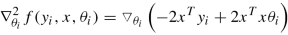(7.35)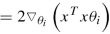(7.36)
\displaystyle \begin{aligned} & = 2 \left(x^Tx\right)^T \end{aligned}
(7.37)
\displaystyle \begin{aligned} & = 2 x^Tx. \end{aligned}
(7.38)

The Hessian matrix $$\nabla _{\theta _i}^2 f(y_i,x,\theta _i)$$ is positive semidefinite based on Theorem 2. Then, we have f(y i, x, θ i) is convex in θ i based on Theorem 1. The objective f(y, x, θ) is convex with respect to θ, because the sum of convex functions, $$\sum _{i=1}^{N}f(y_i,x,\theta _i)$$, is still a convex function.

### 7.1.3 The Objective Is Convex with Respect to x

The objective function for sample i is

\displaystyle \begin{aligned} f(y_i, x, \theta_i) = y_i^Ty_i - 2y_i^Tx\theta_i + \theta_i^Tx^Tx\theta_i. \end{aligned}
(7.39)

We cast x as a vector $$\bar {x}$$, which is formed by stacking the columns of x in order. We rewrite the objective function as

\displaystyle \begin{aligned} f(y_i, \bar{x}, \theta_i)=a_i - 2b_i^T\bar{x} + \bar{x}^TC_i\bar{x}. \end{aligned}
(7.40)

The coefficients are formed such that

\displaystyle \begin{aligned} a & = y_i^Ty_i, \end{aligned}
(7.41)
\displaystyle \begin{aligned} b_i^T\bar{x} &= y_i^Tx\theta_i, \end{aligned}
(7.42)
\displaystyle \begin{aligned} \bar{x}^TC_i\bar{x} &=\theta_i^Tx^Tx\theta_i. \end{aligned}
(7.43)

The linear coefficient matrix is the KM × 1 vector

\displaystyle \begin{aligned} b_i=[y_i\theta_{1i},\ldots,y_i\theta_{Ki}] \end{aligned}
(7.44)

The quadratic coefficient is the KM × KM and block matrix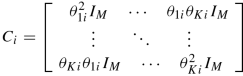(7.45)

The gradient with respect to $$\bar {x}$$

\displaystyle \begin{aligned} \nabla_{\bar{x}}f(y_i,\bar{x},\theta_i)& = -2b_i + 2C_i\bar{x}. \end{aligned}
(7.46)

Take second derivative to get Hessian matrix,

\displaystyle \begin{aligned} \nabla_{\bar{x}^2}f(y_i,\bar{x},\theta_i)& = 2C_i^T \end{aligned}
(7.47)
\displaystyle \begin{aligned} & = 2\left(\theta_i\theta_i^T\right)^T \end{aligned}
(7.48)
\displaystyle \begin{aligned} & = 2\left(\theta_i^T\right)^T\left(\theta_i^T\right). \end{aligned}
(7.49)

The Hessian matrix $$\nabla _{\bar {x}}^2 f(y_i,\bar {x},\theta _i)$$ is positive semidefinite based on Theorem 2. Then, we have $$f(y_i,\bar {x},\theta _i)$$ is convex in $$\bar {x}$$ based on Theorem 1. The objective f(y, x, θ) is convex with respect to x, because the sum of convex functions, $$\sum _{i=1}^{N}f(y_i,x,\theta _i)$$, is still a convex function.

The objective is biconvex with respect to both x and θ. Thus, we have a biconvex optimization problem based on the proof of biconvexity of the constraints and the objective.

### Appendix 3: A-Star Search Algorithm

In this procedure, first we remove all the duplicate and all-zero coefficients hyperplanes to get unique hyperplanes. Then we start from a specific region r and put it into a open set. Open set is used to maintain a region list which need to be explored. Each time we pick one region from the open set to find adjacent regions. Once finishing the step of finding adjacent regions, region r will be moved into a closed set. Closed set is used to maintain a region list which already be explored. Also, if the adjacent region is a newly found one, it also need to be put into the open set for exploring. Finally, once the open set is empty, regions in the closed set are all the unique regions, and the number of the unique regions is the length of the closed set. This procedure begins from one region and expands to all the neighbors until no new neighbor is existed.

The overview of the A-star search algorithm to identify unique regions is shown in Algorithm 1.

### Algorithm 1 A-star Search Algorithm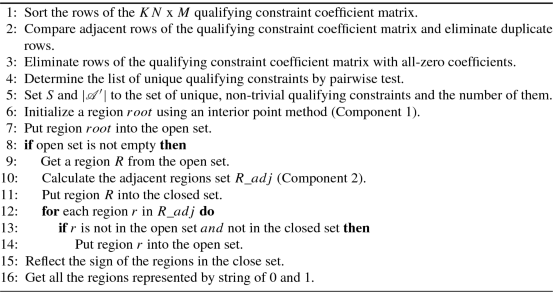### 7.1.1 Hyperplane Filtering

Assuming there are two different hyperplanes H i and H j represented by $$A_i=\left \{a_{i,0},\ldots ,a_{i,MK}\right \}$$ and $$A_j=\left \{a_{j,0},\ldots ,a_{j,MK}\right \}$$. We take these two hyperplanes duplicated when

\displaystyle \begin{aligned} \frac{a_{i,0}}{a_{j,0}}=\frac{a_{i,1}}{a_{j,1}}=\ldots=\frac{a_{i,MK}}{a_{j,MK}}=\frac{\sum_{l=0}^{MK}a_{i,l}}{\sum_{l=0}^{MK}a_{j,l}}, a_{j,l}!=0 \end{aligned}
(7.50)

This can be converted to

\displaystyle \begin{aligned} \left| \sum_{l=0}^{MK}a_{i,l}\cdot a_{j,n}-\sum_{l=0}^{MK}a_{j,l}\cdot a_{i,n} \right| \leq \tau, \forall \ n \epsilon [0,MK] \end{aligned}
(7.51)

where threshold τ is a very small positive value.

We eliminate a hyperplane H i represented by $$A_i=\left \{a_{i,0},\ldots ,a_{i,MK}\right \}$$ from hyperplane arrangement $${\mathcal {A}}$$ if the coefficients of A i are all zero,

\displaystyle \begin{aligned} \begin{array}{rcl} |a_{i,j}|\leqslant \tau &\displaystyle \text{for all}\ a_{i,j} \in A_i\ \text{and}\ j\in [0,MK] \end{array} \end{aligned}

The arrangement $${\mathcal {A}}^\prime$$ is the reduced arrangement and A x = b are the equations of unique hyperplanes.

### 7.1.2 Interior Point Method

An interior point is found by solving the following optimization problem: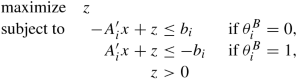(7.52)

### Algorithm 2 Interior Point Method (Component 1)### Algorithm 3 Get Adjacent Regions (Component 2)## Rights and permissions

Reprints and Permissions